Announcements
MAINTENANCE ALERT: Dec. 12th starting 9 AM CET. Community will be read-only. GET DETAILS
cancel
Showing results for
Did you mean: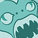Partner - Contributor III

## Accumulated Value in pivot with n dimensions, where value is reset when dim 1 changes

Hi.

In a pivot table I need to Acc. a QTY per Item over date and Trans Type.

Hovever, I have several additional dimensions that are conditional and can be selected to be shown/hidden. An Aggr with all dimensions is therefore not an option!

There are three trans types:

Stock, Sales Order and Purchase. Stock always has to be first value.

At first I tried:

RangeSum(Above(TOTAL Sum(QTY), 0, RowNo(TOTAL)))

The problem was of course that the value was just accumulated disregarding the item.

The value needs to be reset when the Item changes and then start the accumulation from the "Stock" value.

I tried to do an if that would determine if we had a new item and therefore my final guess was this:

If(Above(TOTAL Item) = Item,
RangeSum(Above(TOTAL Sum(QTY), 0, RowNo(TOTAL))),
Sum(QTY)
)

The result is that the first line for the new item works but then the accumulation begins from the top. How do I force the calculation with the Item?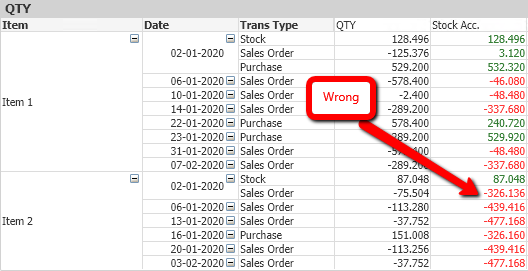Labels (3)

• ### Total

1 Solution

Accepted SolutionsPartner - Contributor III
Author

I ended up solving it quite simply this way in the chart expression:

if(Item<>Above(TOTAL Item),
Sum(QTY),
Sum(QTY) + Above(TOTAL [Qty Acc.])) // Where [Qty Acc.] is the header name.

8 RepliesMVP

Try this

If(Item=Above(TOTAL Item), RangeSum(Above(TOTAL Sum(QTY), 0, RowNo(TOTAL))), Sum(QTY))

Before develop something, think If placed (The Right information | To the right people | At the Right time | In the Right place | With the Right context)Partner - Contributor III
Author

Hi Anil.

Thank you for your reply.  Your solution seems to work within the "Trans Type" only. Whenever we get to a new date we start from the first value: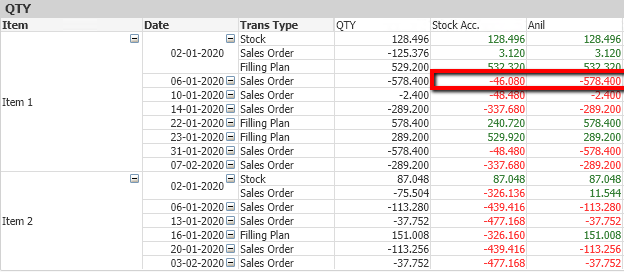MVP

Try to use Another condition for Date field also, If that is the case?

If(Item=Above(TOTAL Item) or Date=Above(TOTAL Date), RangeSum(Above(Sum(QTY), 0, RowNo(TOTAL))), Sum(QTY))

Before develop something, think If placed (The Right information | To the right people | At the Right time | In the Right place | With the Right context)Partner - Contributor III
Author

Hi Anil.

The result is unfortunately the same. No values changed compared to the last solution.MVP

Can you please post some rows and provide the expected result, May be try with

If(Item=Above(TOTAL Item), If(Date=Above(TOTAL Date), RangeSum(Above(Sum(QTY), 0, RowNo(TOTAL))), Sum(QTY)))

Before develop something, think If placed (The Right information | To the right people | At the Right time | In the Right place | With the Right context)Partner - Contributor III
Author

Hi again Anil.

Thank you for another answer. Sorry, but now I get this result:Partner - Contributor III
Author

Let me try again: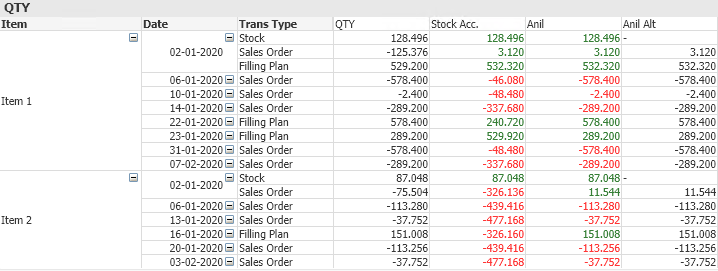Your alternative solution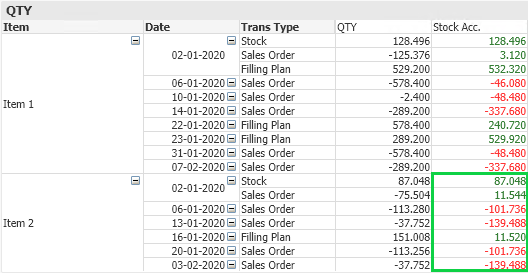The solution I am looking forPartner - Contributor III
Author

I ended up solving it quite simply this way in the chart expression:

if(Item<>Above(TOTAL Item),
Sum(QTY),
Sum(QTY) + Above(TOTAL [Qty Acc.])) // Where [Qty Acc.] is the header name.Community Browser